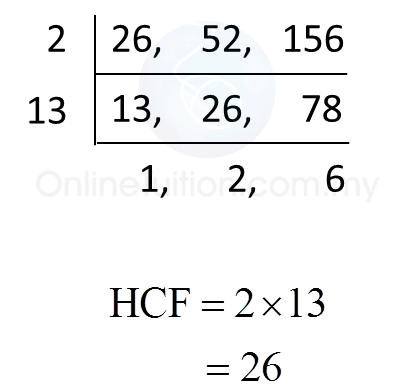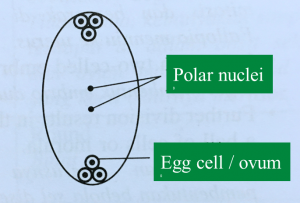# 2.2.4 Number Patterns and Sequences, PT3 Practice

 [adinserter block="3"]       
Question 16:
 State the first two common multiples of 3, 5 and 6.
 
 Solution:
 
Multiples of 3 = 3, 6, 9, 12, 15, 18, 21, 24, 27, 30, 33, 36, 39, 42, 45, 48, 51, 54, 57, 60, …
 Multiples of 5 = 5, 10, 15, 20, 25, 30, 35, 40, 45, 50, 55, 60, …
 Multiples of 6 = 6, 12, 18, 24, 30, 36, 42, 48, 54, 60, …
 
 The first two common multiples of 3, 5 and 6 are 30 and 60.
 
 
 
 [adinserter block="3"]       
Question 17:
 Find the lowest common multiple (LCM) of 4, 8 and 24.
 
 Solution:[adinserter block="3"]       
Question 18:
 Find the lowest common multiple (LCM) of 8, 12 and 15.
 

 Solution:[adinserter block="3"]   
Question 19:
 Find the highest common factor (HCF) of 26, 52 and 156.
 

 Solution:[adinserter block="3"]   
Question 20:
 Given that (m + 5) is the highest common factor of 81 and 108, find the value of m.
 

 Solution:# 2.2.3 Number Patterns and Sequences, PT3 Practice

Question 11:
State all the prime factors of 156.

Solution:

Question 12:
State all the prime factors of 182.

Solution:

# 4.9.5 Sexual Reproduction in Flowering Plants (Structured Question 1 & 2)

Question 1:
Diagram below shows a longitudinal section of the reproductive parts of a flower during fertilization.(a)
On the Diagram, name the structures J, K, L and M.

(b)(i)
In the space below, draw a section through the ovule, showing all the cells in M.
(b)(ii) What is the significance of having two K structures in the fertilization?

(c)
In the Diagram, the structure Y has to be kept dormant for future research purposes.
(i) Explain how Y can be prevented from germinating.
(ii) If Y is to be germinated, suggest one method to stimulate the germination of Y.

(a)
J : Pollen tube
K : Male gamete
L : Ovary
M : Embryo sac

(b)(i)(b)(ii)
- One K/ male gamete fertilizes an egg cell to form a diploid zygote.
- One more K fuses with two polar nuclei to form a triploid zygote.

(c)(i)
- Keep Y in a dry place
- Moisture initiates germination

(c)(ii)
Spraying a sugary solution onto Y